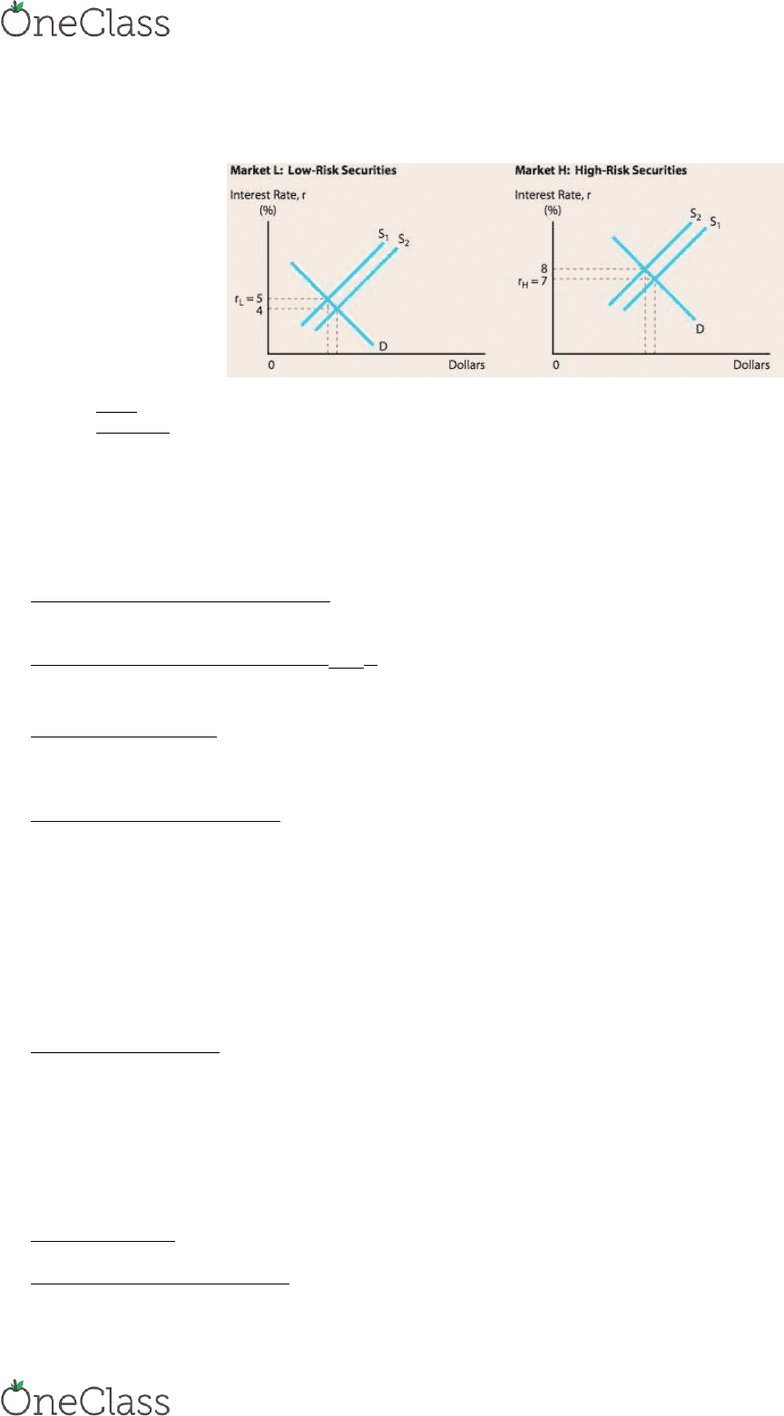Textbook Notes (280,000)
US (110,000)
NU (1,000)
FINA (60)
Chapter 6

# FINA 2201 Chapter Notes - Chapter 6: Investment, Yield Curve, United States Treasury Security

Department
Finance & Insurance
Course Code
FINA 2201
Professor
Ma Linlin
Chapter
6

This preview shows half of the first page. to view the full 3 pages of the document.FINA 2201 Chapter 6: Interest Rates
1
The cost of money
The interest rate is the price that lenders receive and borrows pay for debt capital.
o Cost of Debt
o How should price be determined?
o Supply and Demand for capital
Risk: In a financial market context, the chance that an investment will provide a low or negative return.
Inflation: The amount by which prices increase over time
o Inflation has a major impact on interest rates because it erodes the real value of what you receive in the
future.
Quoted interest rate = r = r* + IP + DRP + LP + MRP
= RRF + DRP + LP + MRP
Real Risk-Free Rate of Interest (r*):The rate of interest that would exist on
default-free U.S. Treasury securities if no inflation were expected.
Nominal (Quoted) Risk- Free Rate, (rRF):The rate of interest on a security that is free of all risk; rRF is proxied by the T-
bill rate or the T-bond rate. rRF includes an inflation premium.
Inflation Premium (IP): A premium equal to expected inflation that investors add to the real risk-free rate of return (the
average expected inflation rate over the life of the security)
Note that it is the expected value, not the rate experience in the past.
Default Risk Premium (DRP):The difference between the interest rate on a U.S. Treasury bond and a corporate bond of
equal maturity and marketability. (The risk that the borrower will default)
The greater the bond’s risk of default, the higher the interest rate
o Gov. Bonds versus Corporate Bonds
Bond Ratings:
o AAA: extremely strong capacity to meet its financial commitments.
o BBB: adequate capacity to meet its financial commitments.
o CCC: dependent upon favorable business, financial, and economic conditions to meet its financial
commitments.
Liquidity Premium (LP): A premium added to the equilibrium interest rate on a security if that security cannot be converted
to cash on short notice and at close to its “fair market value.”
A liquid asset can be converted to cash quickly at a “fair market value”
Investors prefer assets that are liquid
o Real assets versus financial assets
Liquidity premium can be measured by
Interest Rate Risk: The risk of capital losses to which investors are exposed because of changing interest rates.
Bonds typically pay a fixed rate of interest and principal when a bond matures.
Available maturities range from 30 days to 30 years.
Maturity Risk is the potential for interest rates to change while your money is tied up
r*: real risk-free rate of interest
rRF: interest rates on U.S. treasure bills